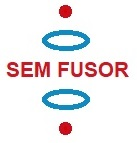A new idea to obtain clean fusion energy

15. Confinement of positive ions and electrons with a static electric and magnetic field

Experiments with the simulation program: simplified SEM fusor design with only 2 rings

Back to main page

 Fig.1. Exp. 15.1 Only two negatively charged rings and a magnetic field of 0,3 T. Cross section with the electric field displayed: arrow is direction, color is strenght (the redder, the stronger). Fig.2. Exp. 15.1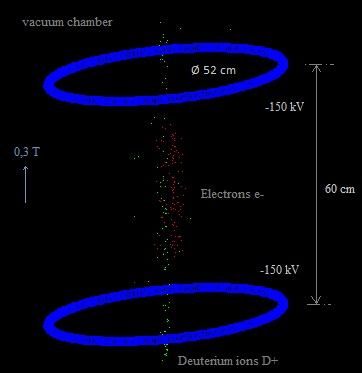The magnetic field is relatively weak. The particles stay more or less confined. The maximum vertical speed of the D+ ions is about 2,2 E6 m/s (I checked this in the simulation). This is about the speed necessary for fusion. Because in the simulation there are so few particles, no colisions take place. In reality there would be collisions and some particles also will obtain high speeds in the lateral direction. To stop them from escaping the magnetic field should be stronger, perhaps. This configuration with only two rings is quite simpler, but the one with three rings and two spheres does confine the particles better. ----------------- Fig.3. Exp. 15.2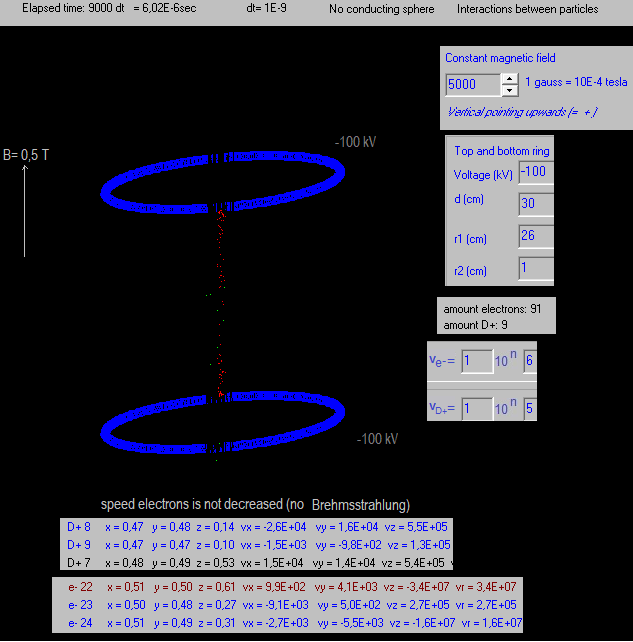Less voltage, a little more magnetic field. The D+ ions are injected a little below the lower ring, the electrons are injected a little above the lower ring, more or less vertically. chcc:=1; electron[i].m:=chcc*me; {3E20 is the amount of D+ in the vacuum chamber if p=3E-2 torr}; electron[i].vx:=0 + (-0.5 + random)*ve/1000; electron[i].vy:=0 + (-0.5 + random)*ve/1000; electron[i].vz:=0 + (-0.5 + random)*ve/1000+vie; electron[i].q:=chcc*-qe{*3E8}; {3E20 is the amount of D+ in the vacuum chamber if p=3E-2 torr} electron[i].x:=0.50+ ( - 0.5 + random) /100; {so they start not in exactly the same point}; electron[i].y:=0.50+ ( - 0.5 + random) /100; {so they start not in exactly the same point}; electron[i].z:= 0.5-dr+r2r+0.06; //0.35+ ( - 0.5 + random) /100; {so they start not in exactly the same point}; When the D+ ions are more or less cross the centre region, their vertical speeds are a little bit larger than their initial speeds (about 5,5E+5). ---------------------- Fig.4. Exp. 15.3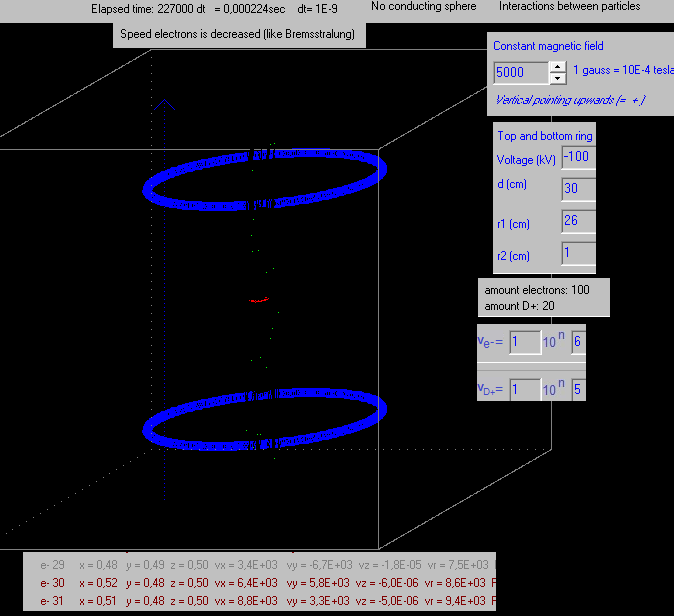The speed of the electrons is slowly reduced in the simulation (to simulate Bremsstrahlung). if DecreaseSpeedElectrons=true then begin if t=1 then {if electron} begin vx:=vx*0.90; vy:=vy*0.90; vz:=vz*0.90; end; end; It is curious that the speed of the electrons in the vertical direction (z) is a lot more reduced that the speed in the horizontal direction (x, y).  They were generated with small random speeds in the x-y direction.   Fig.5. Exp. 15.3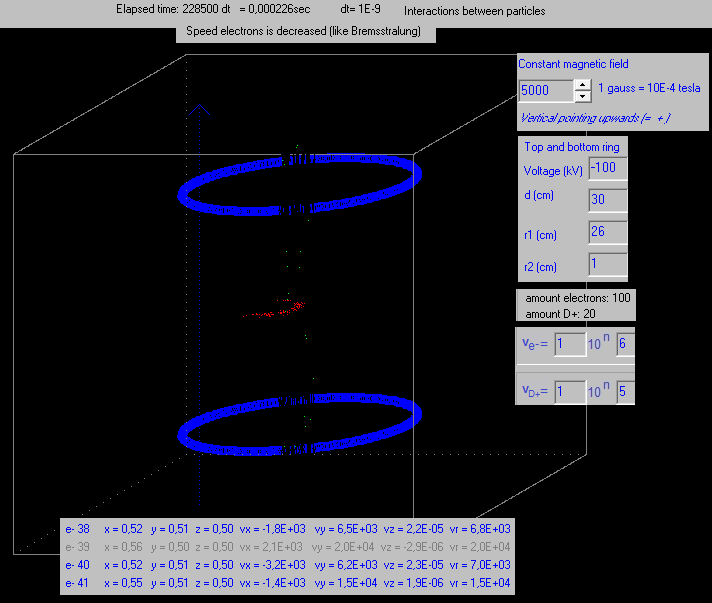The same experiment, but a little later in time. The electrons have spread out a little more in the x-y plane.   Fig.6. Exp. 15.3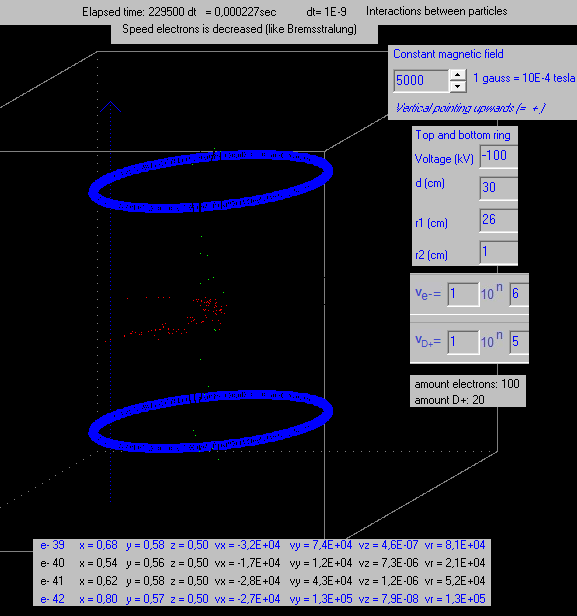The same experiment, again a little later in time. The electrons are even more spread out in the x-y plane. But why? They still have a speed vx and vy, so the magnetic field should impede them to move outwards.. B = m.v / ( r . q) With a horizontal speed of  3.103 m/s  ->  r = m.v / (B .q) = 9.10 ×10−31  . 3.103   / (0,5 . 1.6021×10−19 ) = 3,38 .10−8 m   Fig.6. Exp. 15.3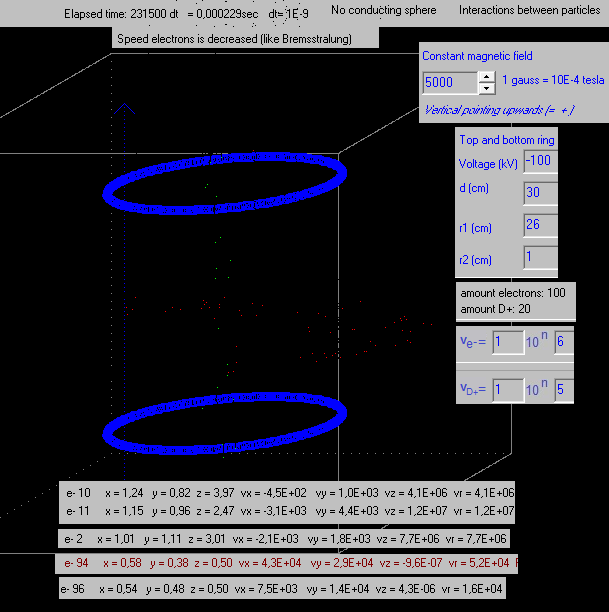The same experiment, again a little later in time. The electrons have even more spread out in the x-y plane and some have escaped. They seem to escape when they are at a certain distance from the centre vertical axis. Exp. 15.3 fig. 7 screenshot.png (part of the screen is made grey, to see the field lines better) When the electrons escape: as seen in the screenshot, at some distance from the vertical centre axis, the electric field lines become horizontal. At a sufficient large distance from the vertical centre axis, the two charged rings can also be considered as a point charge in the centre, and in this way you can understand why they are repelled upwards or downwards. Sidewards they do not go, because of the vertical magnetic field.   Fig. 8. Exp. 15.4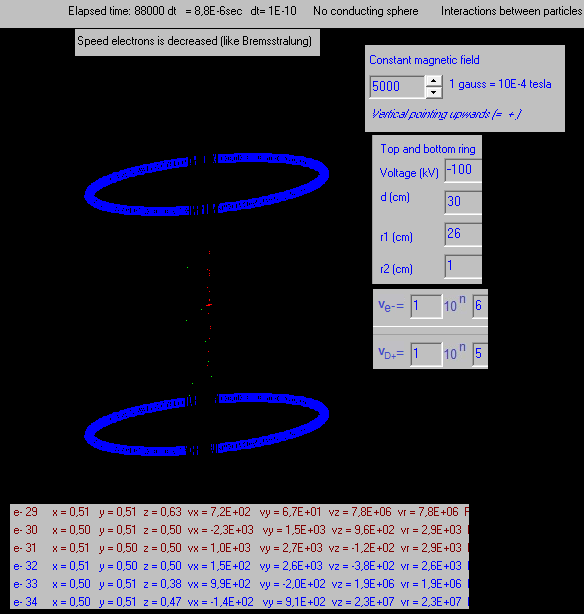The speeds of the electrons is reduced, but only as long no one of the vx, vy and vz components are not smaller than 1E3 m/s: if (vx>1E3) and (vy>1E3) and (vz>1E3) then Begin vx:=vx*0.90; vy:=vy*0.90; vz:=vz*0.90; end; It seems to be that at least one of the speeds vx, vy or vz < 1E3, so the speeds are not further decreased by the program. But why in 15.4 the electrons do now not spread out, and in experiment 15.3 yes they do? And why the vz component of the speed of the electrons in experiment 15.3 becomes very small (almost zero), and in experiment 15.2 (without Bremsstrahlung) not ? When we keep reducing the speeds in the program, the vz component becomes almost zero, but the vx and vy components keep some small value (about 1E2, 1E3 m/s): Why is this so?   Fig. 9. Exp. 15.5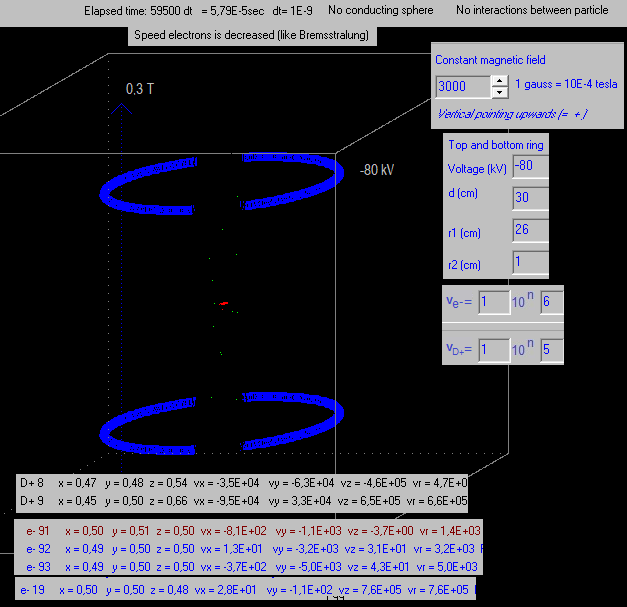if DecreaseSpeedElectrons=true then begin if t=1 then begin if (vx>1E2) and (vy>1E2) and (vz>1E2) then Begin vx:=vx*0.90; vy:=vy*0.90; vz:=vz*0.90; end; end; end; The electrons keep well confined in the centre area. But there are only few (not 1020 ).   Again the speed component vz is a lot more reduced than the components vx and vy. I tried out: if (vx>1E2) and (vy>1E2) and (vz>1E2) then Begin vx:=vx*0.90; vy:=vy*0.90;  {without vz decreasing} end; and the component  vz did not change. So the vz is reduced by the program, and not because vx and vy are reduced.   Fig. 10. Exp. 15.6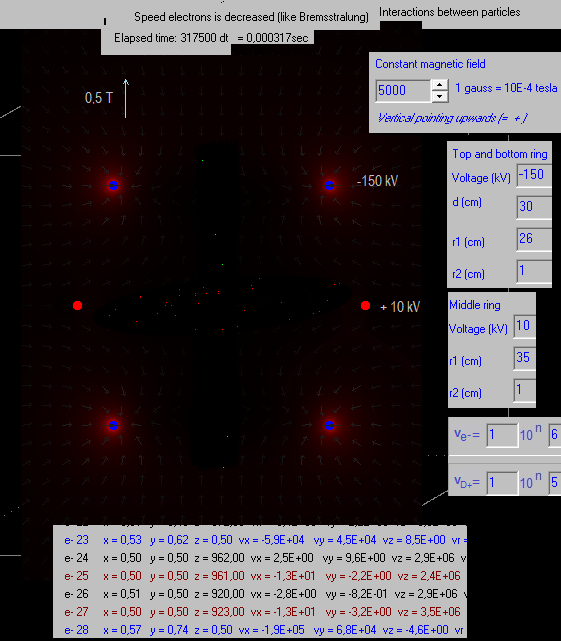The mass and the charge of the electrons is multiplied with 108 in the above experiment. The particles do interact amongst each other. The speed of the electrons is decreased: Begin vx:=vx*0.90; vy:=vy*0.90; vz:=vz*0.90; end; Fig. 10. Exp. 15.6 screenshot1.png Fig. 10. Exp. 15.6 screenshot2.png Fig. 10. Exp. 15.6 screenshot3.png The electrons escape.   Fig. 11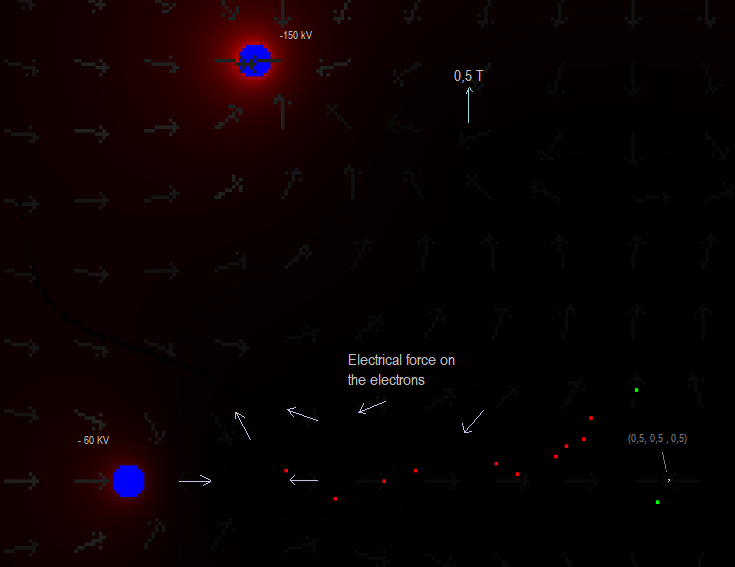Charging the middle ring with a negative charge, will not stop the electrons from escaping, see the image above (part of the cross section has been enlarged). The charge and mass of the electrons are the same as in 15.5 (augmented with 108 ). The electrons escape.   Fig.12 Exp. 15.7.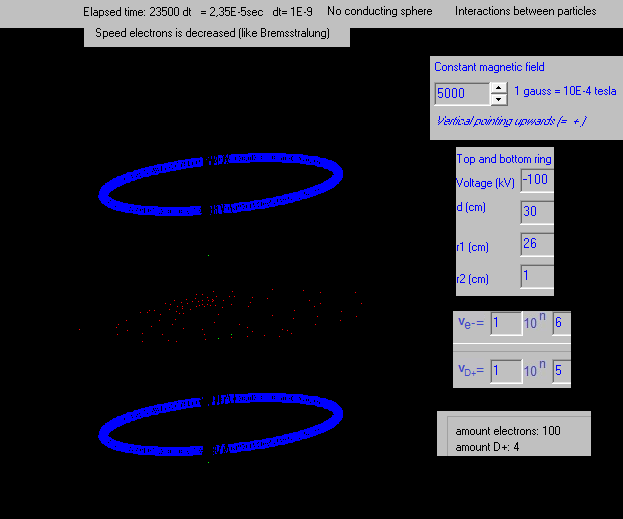Exp.15.7 .mp4 The mass and the charge of the electrons is multiplied with 108 in the above experiment. The speed of the electrons is decreased: Begin vx:=vx*0.90; vy:=vy*0.90; vz:=vz*0.90; end; The electrons spread out, due to the augmented charge (x 108 ). Interactions between the particles is on. (Coulomb and Bios-Savart). ------------------------- Let´s create a negative charge in the centre region. I was thinking to generate the D+ ions random, but then many of them will colide with the negative charged rings up and down, and this will cause (huge?) losses. It seems to be that the best is to inject both the D+ and the electrons. In the link below an electron gun is sold with an energy range: 1 eV to 100 keV and a beam current range: 1 nA to 20 mA (or even 50 mA) https://www.kimballphysics.com/    (1 A = 6.242×1018 electrons/s)   Let´s take 1 mA -> 1.10-3 A = 1.10-3 C/s -> 1.10-3 C/s / (1,6.10-19 C) = 6,3. 1015   electrons/s It seems therefore plausible to have an electron gun that produces about 6.3. 1015    electrons/ s ( 1 electron/1,59 10-16  s)   If the energy of an electron is 1 eV, then:   ½ meVe2 = 1 eV = 1,602.10-19 J  => Ve = √ (2 .  1,602.10-19 / (9,1095 . 10-31) ) = 5,931. 105  m/s   It seems to be possible to give them speeds of 1 . 106  m/s (as in the simulation program).   How much charge we have after one minute:  60 x 6,3. 1015   electrons =  3,8. 1017    electrons ->   3,8 . 1017  . 1,6.10-19 C =  6,0 10-2 C   In the simulation program we will use the middle ring to simulate, quite approximated, a negative charge in the centre region. How much negative voltage we must give it to be equivalent with  6,0 10-2 C ?     According the simulation program, for a charged ring, if V = 100 kV then Q = 8,93.10-7 C   6,0 . 10-2 C / ( 8,93. 10-7 C ) = 6,8 . 104 6,8  104  x 100 kV = 6,8 . 106 V = 6800  kV   That would be a huge acumulation of charge. Anyway the electron gun´s capacity will not be a problem if we want to create an accumulation of negative charge.   Let´s take in the simulation program a middle ring with -20 kV.   With how many electrons this will correspond? 100 kV   -> 8,93.10-7 C 20 kV -> 1,8 . 10-7 C  -> 1,12 1012 electrons   To have an electric equilibrium we should have the same amount of D+ ions.   How much fusion energy we could get from 1,12 1012  D+ ions?   (see also Droom11.html# )   As 2 D+ ions fuse, there is liberated per D+ ion  = 2,9E-13 J   (3,7/2 MeV = 1,8E6 . 1,6E-19 J)   1,12 1012 D+ ions correspond to 1,12 1012  x 2,9E-13 J  = 0,33 J.   8,78E10 J =2283 ltr diesel   1 m3 diesel = 41 Gigajoule 1 joule = 2,4 .10-8 ltr diesel   Let´s say we want to produce in a Sem Fusor (0,5 m diameter, 1 m high + magnet and equipment) the amount of energy that is the equivalent of  1000 ltr diesel in one hour. 1000 ltr diesel = 4,17 . 1010 joule    ->  4,17 . 1010 / 3600) = 11,6 . 106 joule/s = 11,6 MW  4,17 . 1010 joule  / (  2,9E-13 J  ) = 1,4 . 1023  D+ ions. massa deuterium ion D+ = 2 . 1,67E-27 kg = 3,34E-27 kg 1,4 . 1023  D+ ions x 3,34E-27 kg  = 4,8 . 10-4 kg D+ ion = 0,48 gram deuterium gas   Suppose we inject this quantity of D+ ions into the vacuum chamber. To mantain the electric equilibrium we should inject the same amount of electrons into the chamber.   1,4 . 1023 electrons .  1,6.10-19 C  = 22400 C / hour = 6,2 C / s = 6,2 A   There are telephone chargers that deliver about 3 A, a micro wave about 8 A.   To get the energy of 1000 ltr diesel in one hour we need 0,48 gram deuterium gas (if it fuses completely). This is not so much.   Control: massa deuterium ion = 2 . 1,67E-27 kg = 3,34 E-27 kg 0,48 gram deuterium -> 0,48 E-3 / 3,34 E-27 = 1,44 E23 ions  -> charge =  1,44 E23 .  1,602.10-19 = 2,3 E 4 C 2,3 E 4 C / 3600 = 6,4 A   Just for curiosity. If we divide the charge of 0,48 gram deuterium gas into two halves, and place them at a distance of 0,5 mtr from each other, consider them as point charges, what force do they exert on each other?   Fig. 13.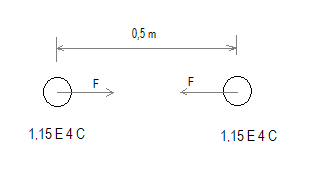F = 9E9 1,15 E4 . 1,15 E4 / 0,52  = 4,8 E18 N, which is huge!!  (4,8 E14 ton force) The amount of "electricity" in half a gram of gas is incredible..     Let´s charge the middle ring with 1 V.   I have simulated this, 1 V is very little, but almost no effect on the D+ ions.     Fig. 14. Exp. 15.8.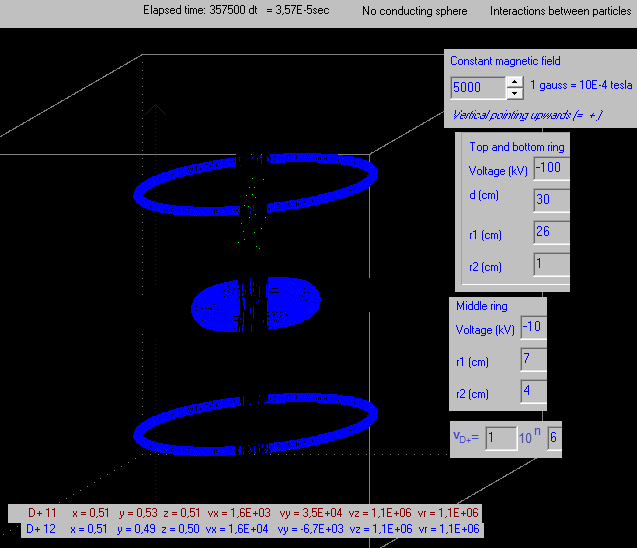The middle ring is charged with -10 kV in the simulation. This should be our cloud of electrons.   hydrogen[i].z:= 0.50 -dr+r2r+0.05; -> the D+ ions are injected a little bit above the lower ring. The electrons cannot be injected below the lower ring, because they will then escape downwards.   -10 kV corresponds with  1,12 1012 / 2  electrons =  0,6. 1012 electrons ( see #Voltage to amount of electrons )   With a 1 mA electron gun (1 E-3 C/s) this will produce:  ( 1 . 10-3  C/s)  / (1,6.10-19 C/electron) = 6,25 . 1015 electrons/s We could use an electron gun with a lot less capacity, if we want to create an electron cloud with a charge equal, more or less, to the charged middle ring in the simulation.   The time it takes to produce this amount of electrones with this electron gun:  0,6. 1012 /  6,25 . 1015  =  9,6 . 10-5 s   If we fuse an equal amount of D+ ions in this time, we´ll get: 0.6E12  . 2,9E-13 J =  0,174  J  (see  Droom11.html# ) -> power = 0,174  J / (  9,6E-5 s ) = 1,9 E 3 J/s (watt) = 1 kW (the same power as an electrical heater)   ---------------------- Conclusion, so far:   We want to inject the deuterium ions. To maintain electric equilibrium, we also have to inject electrons. If all injected deuterium (0,5 gram/hrs) fuse, we would obtain a power of 11,6 MW with a beam of D+ ions and electrons corresponding with a current of 6,2 A.   If we generate random the D+ ions (not inject them, but just a little D2 gas inside the vacuum chamber), probably many D+ ions will collide against the negatively charged rings and cause losses. But if some D+ ions keep trapped and fuse, then this would be also quite an achievement!   Doubts: How much of the injected deuterium will fuse? Will the electrons form a negative cloud in the centre, a kind of virtual negative electrode? Can an electro gun and a "deuterium ion gun" be made with a capacity of about 6 A?   It should be interesting to do real systematic experiments.20 February 2014 ..     by  Rinze Joustra        www.valgetal.com